# QA

## Overview

The Functional Imaging Federated Informatics Research Environment (FBIRN) QA metrics are generated on weekly basis to monitor stability of the scanner. This is a multicenter fMRI protocol executed on an agar Phantom using the 32- channel head coil. Details of the protocol can be found at the end of each report. Longitudinal results of mean ghost percentage, signal-to-fluctuation noise ratio (SFNR), and signal-to-noise ratio (SNR) recorded and reported weekly. Abnormalities in any of the metrics may indicate instability of some hardware component, and such abnormalities are further investigated. In addition, Siemens customer QA tool is run to check coil elements of the head coil weekly and body coil monthly or whenever needed.

The acceptable mean ghost percentage threshold is less than 3% percent (ref 2). The first QA conducted on the DBIC's Siemens Prisma Magnetom was September 12, 2016.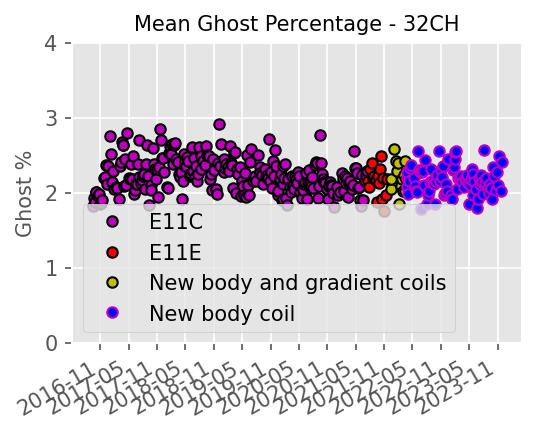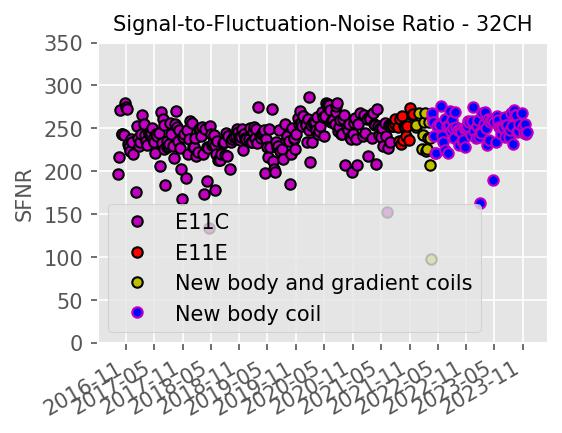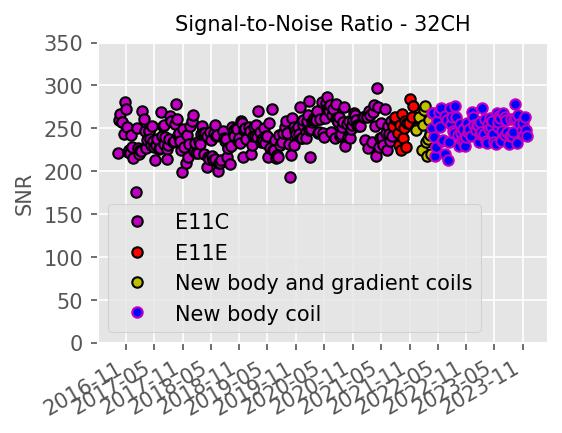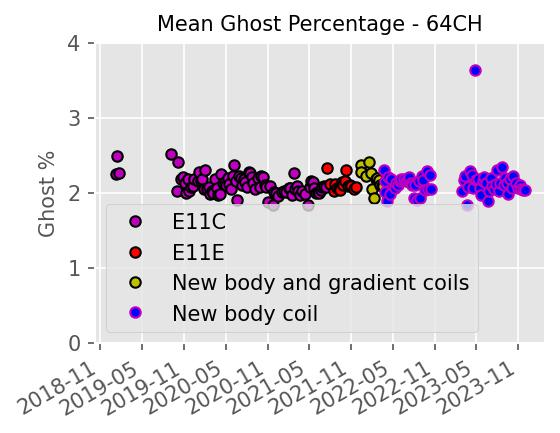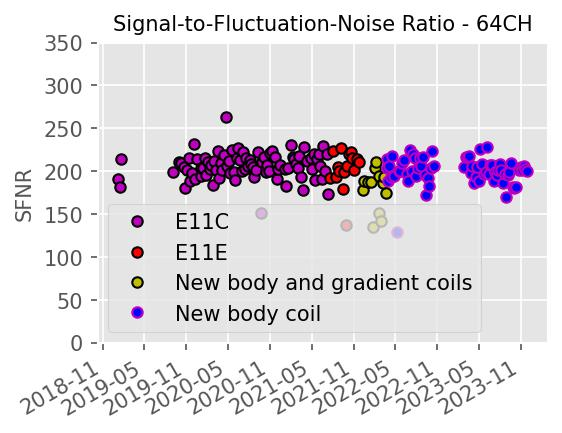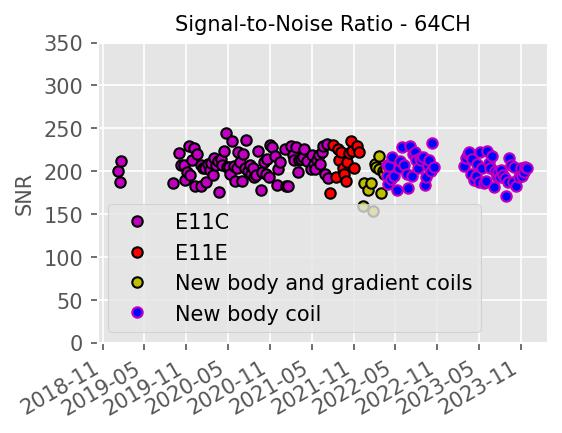## Weekly qa metrics

Below are definitions of the metrics found on the DBIC's weekly QA report. Definitions have been adopted from the original authors.

• Signal image - the mean signal across time
• Signal summary value - the mean across the ROI of the signal image
• Standard Deviation Image (Temporal Fluctuation Noise Image) - voxel by voxel residuals of second-order polynomial fit across
• SNR summary value - the signal summary value ('mean') divided by the standard deviation across the ROI of the static spatial noise image
• SFNR summary value - the mean across the ROI of the signal image divided by the temporal fluctuation image
• SNR Image
• SFNR Image
• Percent fluctuation - 100*std/msi where 'std' is a summary value calculated as the standard deviation of the residuals of a second-0rder polynomial fit of the time series and 'msi' is mean signal intensity of the time-series.
• Drift - 100*(maxroi-minroi)/msi where 'maxroi' and 'minroi' are the maximum and minimum values of the mean raw signal over the ROI.
• Driftfit - 100*(maxfit-minfit)/msi where 'maxfit' and 'minfit' are the maximum and minimum values of the fit.
• Fourier Analysis (Magnitude Spectrum) - the frequency spectrum resulting from a Fourier analysis of the residual data.
• Radius of Decorrelation (RDC) - a measure of the size of ROI at which statistical independence of the voxels is lost.
• Smoothness (Full-width Half-maximum) - calculated using the AFNI tool 3dFWHMx
• Center of mass - a simple mean intensity of each volume, with each voxel's value weighted by its index in the X, Y, or Z direction.
• Odd-Even Difference Static Noise Image
• Mean Ghost Percentage - 100*meanGhost/meanSignal. The ghost metrics are calculated for each volume by taking a dilated mask ("original mask") of the data, and shifting it by N/2 voxels in the appropriate axis to create a "ghost mask". The mean intensities of those voxels in the original mask and not in the ghost mask ("meanSignal") and of voxels in the ghost mask and not in the original mask ("meanGhost") are calculated.

References:

1. Friedman L, Glover, GH. Report on a multicenter fMRI quality assurance protocol. J Magn Reson Imaging. 2006a. 23(6): 827-839. [PubMed: 16649196]

2. Acceptance Testing and Quality Assurance Procedures for Magnetic Resonance Imaging Facilities, AAPM Report No. 100